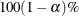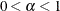# The STDRATE Procedure

### PROC STDRATE Statement

• PROC STDRATE <options>;

Table 107.1 summarizes the options in the PROC STDRATE statement.

Table 107.1: Summary of PROC STDRATE Options

Option

Description

Input Data Sets

Names the SAS data set that contains the study populations

Names the SAS data set that contains the reference population

Standardization Methods

Specifies the method for standardization

Specifies the statistic for standardization

Specifies the test to compare study populations for direct

standardization and Mantel-Haenszel estimation

Displayed Output

Specifies the significance level for confidence intervals

Requests the confidence limits for the standardized estimates

Requests stratum-specific plots

You can specify the following options in the PROC STDRATE statement to compute standardized rates and risks in the procedure. They are listed in alphabetical order.

ALPHA=requests that confidence limits be constructed with confidence level, where. The default is ALPHA=0.05. These confidence limits include confidence limits for the stratum-specific rates or risks, standardized rate and risk, standardized morbidity/mortality ratio, and population attributable rate and risk.

CL=GAMMA <(TYPE=AVERAGE | CONSERVATIVE)> | LOGNORMAL | NONE | NORMAL | POISSON

specifies the method to construct confidence limits for SMR and standardized rate and risk. You can specify the following values for this option:

GAMMA

requests confidence limits based on a gamma distribution for METHOD=DIRECT and METHOD=MH. This value applies only when STAT=RATE. You can specify the TYPE=CONSERVATIVE suboption to request conservative confidence limits that are based on a gamma distribution and were developed by Fay and Feuer (1997), or you can use the default TYPE=AVERAGE suboption to request modified confidence limits proposed by Tiwari, Clegg, and Zou (2006).

LOGNORMAL

requests confidence limits based on a lognormal distribution.

NONE

suppresses construction of confidence limits.

NORMAL

requests confidence limits based on a normal distribution.

POISSON

requests confidence limits based on a Poisson distribution. This value applies only when METHOD=INDIRECT.

The default is CL=NORMAL.

DATA=SAS-data-set

names the required SAS data set that contains the event information in the study populations.

EFFECT <=DIFF | RATIO>

displays a table of the effect estimate and associated confidence limits. This option applies only when METHOD=DIRECT with two study populations and when METHOD=MH, where two study populations are required.

EFFECT and EFFECT=RATIO display a test on the ratio effect of estimates between the study populations, and the EFFECT=DIFF option displays a test on the difference effect.

METHOD= DIRECT | INDIRECT <(AF)> | MH <(AF)>
M= DIRECT | INDIRECT <(AF)> | MH <(AF)>

specifies the required method for standardization. The AF suboption (available only for METHOD=INDIRECT or METHOD=MH) requests the attributable fraction, which measures how much of the excess event rate or risk fraction in the exposed population is attributable to the exposure. This suboption also requests the population attributable fraction, which measures how much of the excess event rate or risk fraction in the total population is attributable to the exposure.

You can specify the following values:

DIRECT

requests direct standardization.

INDIRECT

requests indirect standardization. If you specify the AF suboption, the study population is treated as the exposed population and the reference population is treated as the unexposed population.

MH

requests Mandel-Haenszel estimation. The order of the two study populations is indicated by the ORDER= suboption in the GROUP option in the POPULATION statement. If you specify the AF suboption, the exposed population is identified by the EXPOSED= suboption in the GROUP option in the POPULATION statement. If the EXPOSED= suboption is not specified, then the first study population is treated as the exposed population and the second study population is treated as the unexposed population.

PLOTS <( global-options )> <= plot-request>
PLOTS <( global-options )> <= ( plot-request < …plot-request> ) >

specifies options that control the details of the plots. The default is PLOTS=RATE for STAT=RATE and PLOTS=RISK for STAT=RISK.

You can specify the following global-options:

DISPLAY=INDEX | LEVEL

specifies tick mark values for the strata axis. DISPLAY=LEVEL displays strata levels on the strata axis, and DISPLAY=INDEX displays strata indices of sequential strata identification numbers on the strata axis. The default is DISPLAY=LEVEL.

ONLY

suppresses the default plots and displays only plots that are specifically requested.

STRATUM=HORIZONTAL | VERTICAL

controls the orientation of the plots. STRATUM=VERTICAL places the strata information on the vertical axis, and STRATUM=HORIZONTAL places the strata information on the horizontal axis. The default is STRATUM=VERTICAL.

You can specify the following plot-requests:

ALL

produces all appropriate plots.

DIST | DISTRIBUTION

displays a plot of the proportions for stratum-specific exposed time or sample size.

EFFECT

displays a plot of the stratum-specific effect estimates and associated confidence limits. This option applies only when METHOD=DIRECT with two study populations and when METHOD=MH, where two study populations are required. If the EFFECT=DIFF option is specified, the stratum-specific rate or risk difference effects are displayed. Otherwise, the stratum-specific rate or risk ratio effects are displayed.

NONE

suppresses all plots.

RATE

displays a plot of the stratum-specific rates and associated confidence limits. This option applies only when STAT=RATE. If a confidence limits method is specified in the STATS(CL=) option in the STRATA statement, that method is used to compute the confidence limits. Otherwise, the normal approximation is used.

RISK

displays a plot of the stratum-specific risks and associated confidence limits. This option applies only when STAT=RISK. If a confidence limits method is specified in the STATS(CL=) option in the STRATA statement, that method is used to compute the confidence limits. Otherwise, the normal approximation is used.

SMR

displays a plot of the stratum-specific SMR estimates and associated confidence limits. This option applies only when METHOD=INDIRECT. If a method is specified in the SMR(CL=) option in the STRATA statement, that method is used to compute the confidence limits. Otherwise, the normal approximation is used.

REFDATA=SAS-data-set

names the required SAS data set that contains the event information in the reference population.

STAT=RATE <( MULT =c )>
STAT=RISK

specifies the statistic for standardization. STAT=RATE computes standardized rates, and STAT=RISK computes standardized risks. The default is STAT=RATE.

The MULT= suboption in the STAT=RATE option specifies a power of 10 constant c, and requests that rates per c population-time units be displayed in the output tables and graphics. The default is MULT=100000, which specifies rates per 100,000 population-time units.